From: Lynne If you multiply two polynomials, what can you say about the degree of their product? What exponent applies here? Hi Lynne. There's a short answer to your question if we make some assumptions: the degree of the product is the sum of the degrees of the polynomials that are its factors. For example,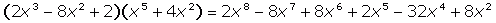So here a single-variable polynomial of degree 3 times another of degree 5 generates a product of degree 8. If you are using single-variable polynomials, then in general, you can write the form of the polynomial of degree n as: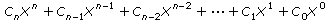where ci are constants. If you multiply that by another single-variable polynomial, for example,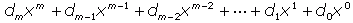then the term of the product with the largest exponent is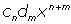or in other words, the degree of the polynomial is (n+m). Note that this all assumes we are dealing with just one variable. If you have more than one variable, the "degree of the polynomial" that is the largest sum of exponents in the terms of the product. For example,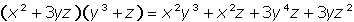has polynomial factors of degree 2 and 3, and the product is of degree 5 because the term x2 y3 is a degree 5 term. Hope this helps, Stephen La Rocque.